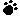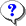Note: You can hover cursor toto find the correct answer.0  (0%)4 Sec

1.As part of a strategy to handle negative risk, a project manager decided to adopt less complex processes, conduct more tests and choose a more stable supplier. What strategy would this be classified as?

A) Acceptance
B) Transference
C) Mitigation
D) AvoidanceYour answer=, Correct answer=, Work Sheet:

2.Rick has just been assigned as the project manager of a project to develop a complex product. The project is in the Define Scope phase. Which of these tools / techniques will not be used by Rick in this phase?

A) Lateral thinking
B) Process analysis
C) Pair wise comparisons
D) Value engineeringYour answer=, Correct answer=, Work Sheet:

3.A number of identified risks occurred early in a project. As a result, most of the project objectives ended up in jeopardy. The project manager decided to present a case to management that the project be closed down. This is an example of:

A) Risk Acceptance
B) Risk Mitigation
C) Risk Avoidance
D) Risk TransferYour answer=, Correct answer=, Work Sheet:

4.The Risk Management plan will usually contain the definitions of risk probability and impact. Which of the following has the highest impact (negative) on a project?

A) A rating of 1.1 on the impact scale
B) A rating of 0.8 on the impact scale
C) A rating of 0 on the impact scale
D) A rating of 0.1 on the impact scaleYour answer=, Correct answer=, Work Sheet:

5.The Iterate Risks project involves determining which risks may impact a project. It is considered an iterative process. What is the frequency of iteration?

A) Every month
B) Every fortnight
C) The frequency of iteration will vary based on the situation
D) Every weekYour answer=, Correct answer=, Work Sheet:

 Click Turn Right Ahead sign for next test